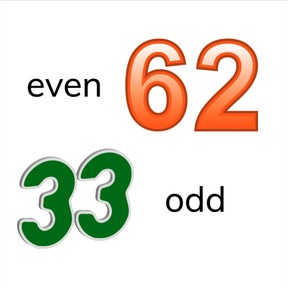Know even and odd numbers to 100

# Know even and odd numbers to 100

Know even and odd numbers to 100

No account needed.8,000 schools use Gynzy92,000 teachers use Gynzy1,600,000 students use Gynzy

## General

Students learn to recognize even and odd numbers to 100. They learn that even numbers are always divisible by 2.

2.OA.C.3

## Relevance

Discuss with students that it is useful to have knowledge of even and odd numbers when you want to know which side of the street a house will be. Explain that house numbers always have an even side and an odd side.

## Introduction

Point at numbers on the interactive whiteboard and ask students to say them aloud. Ask students to skip-count in 2's to reach the given number. Check that students can skip-count in twos by completing the number line.

## Development

Discuss with students that you have to know which number line a number is in to determine if the number is even or odd. Skip-count in 2's. If you start with 0, you will always get an even number, but if you start with 1, you will always get an odd number. For larger numbers you can check if you can divide the number by 2. If the number is divisible by 2, it is even, if it is not, it is odd.

Check that students understand what even and odd numbers are by asking the following questions:
Which house numbers are missing?
Can you explain why 50 is even? And why 77 is odd?

## Guided Practice

Students first practice recognizing numbers, then odd numbers, and finally even and odd numbers.

## Closing

Check that students understand what even and odd numbers are and that they are able to explain why they are even or odd. Finish with a game. Name a random number from 1-100. Students hold up an orange card when the number is even and a blue card for an odd number. You can also use colored pencils instead of cards.

## Teaching Tip

Students might have difficulty with splitting numbers in halves or with skip-counting by 2's. Ask these students to use the number line or other visual representation.

## Instruction materials

Blue and orange cards or colored pencils.

### The online teaching platform for interactive whiteboards and displays in schools

• Save time building lessons

• Manage the classroom more efficiently

• Increase student engagement